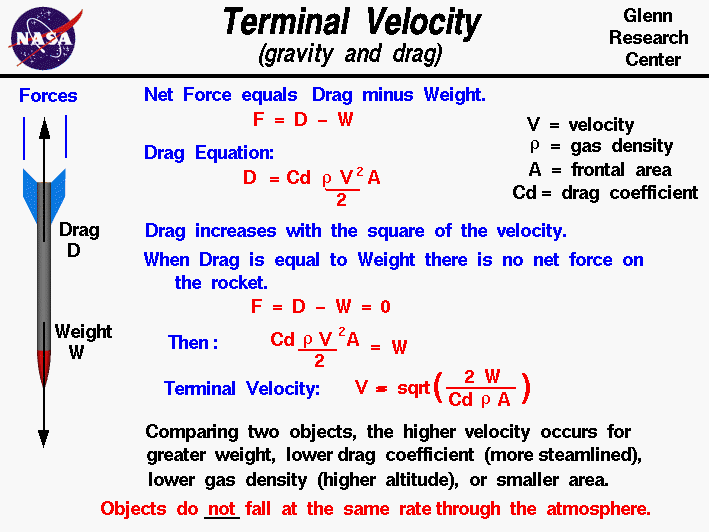+ Text Only Site
+ Non-Flash Version
+ Contact GlennAn object which is falling through the atmosphere is subjected to two external forces. One force is the gravitational force, expressed as the weight of the object. The other force is the air resistance, or drag of the object. If the mass of an object remains constant, the motion of the object can be described by Newton's second law of motion, force F equals mass m times acceleration a:

F = m * a

which can be solved for the acceleration of the object in terms of the net external force and the mass of the object:

a = F / m

Weight and drag are forces which are vector quantities. The net external force F is then equal to the difference of the weight W and the drag D

F = W - D

The acceleration of a falling object then becomes:

a = (W - D) / m

The magnitude of the drag is given by the drag equation. Drag D depends on a drag coefficient Cd, the atmospheric density r, the square of the air velocity V, and some reference area A of the object.

D = Cd * r * V ^2 * A / 2

On the figure at the top, the density is expressed by the Greek symbol "rho". The symbol looks like a script "p". This is the standard symbol used by aeronautical engineers. We are using "r" in the text for ease of translation by interpretive software.

Drag increases with the square of the speed. So as an object falls, we quickly reach conditions where the drag becomes equal to the weight, if the weight is small. When drag is equal to weight, there is no net external force on the object and the vertical acceleration goes to zero. With no acceleration, the object falls at a constant velocity as described by Newton's first law of motion. The constant vertical velocity is called the terminal velocity .

Using algebra, we can determine the value of the terminal velocity. At terminal velocity:

D = W

Cd * r * V ^2 * A / 2 = W

Solving for the vertical velocity V, we obtain the equation

V = sqrt ( (2 * W) / (Cd * r * A)

where sqrt denotes the square root function. Typical values of the drag coefficient are given on a separate slide.

Here's a JavaScipt calculator which will solve the equations presented on this page:

# Terminal Velocity Calculator

Choose planet and units

Enter values for weight, area, and drag coefficient

Enter value for altitude or air density

Press Compute Button

Terminal Velocity

The chemistry of the atmosphere and the gravitational constant of a planet affects the terminal velocity. You select the planet using the choice button at the top left. You can perform the calculations in English (Imperial) or metric units. You must specify the weight or mass of your object. You can choose to input either the weight on Earth, the local weight on the planet, or the mass of the object. Then you must specify the cross sectional area and the drag coefficient. Finally you must specify the atmospheric density. We have included models of the atmospheric density variation with altitude for Earth and Mars in the calculator. When you have the proper test conditions, press the red "Compute" button to calculate the terminal velocity.

You can download your own copy of this calculator for use off line. The program is provided as TermVel.zip. You must save this file on your hard drive and "Extract" the necessary files from TermVel.zip. Click on "Termvcalc.html" to launch your browser and load the program.

When you have gained some experience with the terminal velocity calculator and are familiar with the variables and operation, you can run a simple version of the program on-line. The simple version contains just the calculator and no instructions and it loads faster than the version given above.

Notice In this calculator, you have to specify the drag coefficient. The value of the drag coefficient depends on the shape. of the object and on compressibility effects in the flow. For airflow near and faster than the speed of sound, there is a large increase in the drag coefficient because of the formation of shock waves on the object. So be very careful when interpreting results with large terminal velocities. If your drag coefficient includes compressibility effects, then your answer is correct. If your drag coefficient was determined at low speeds, and the terminal velocity is very high, you are getting the wrong answer because your drag coefficient does not include compressibility effects.

The terminal velocity equation tells us that an object with a large cross-sectional area or a high drag coefficient falls slower than an object with a small area or low drag coefficient. A large flat plate falls slower than a small ball with the same weight. If we have two objects with the same area and drag coefficient, like two identically sized spheres, the lighter object falls slower. This seems to contradict the findings of Galileo that all free falling objects fall at the same rate with equal air resistance. But Galileo's principle only applies in a vacuum, where there is NO air resistance and drag is equal to zero.

We have also developed a simple simulation of a falling object to help you study this interesting physics problem. The program is called DropSim and is available for free at this web site.

Activities:

Guided Tours

Navigation ..Beginner's Guide Home Page+ Inspector General Hotline + Equal Employment Opportunity Data Posted Pursuant to the No Fear Act + Budgets, Strategic Plans and Accountability Reports + Freedom of Information Act + The President's Management Agenda + NASA Privacy Statement, Disclaimer, and Accessibility CertificationEditor: Nancy Hall NASA Official: Nancy Hall Last Updated: Apr 05 2018 + Contact Glenn